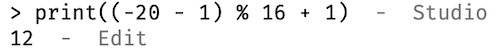# How to get value, which will follow formula y = (x-1) % 16 + 1, but also for negative numbers?

Hello, guys. I’m making mining game. For it I wanted to use chunk system, with chunk size = 16.
Chunk have blocks, starting from 1 and ending with 16th.
To get block chunk position, I used formula:

``````math.floor((Position - 1) / 16) + 1
--------------correct--------------
math.floor((24 - 1) / 16) + 1 = 2
math.floor((0 - 1) / 16) + 1 = 0
math.floor((-16 - 1) / 16) + 1 = -2
``````

To get block position in chunk I used formula:

``````(Position - 1) % 16 + 1
--------------correct--------------
(15 - 1) % 16 + 1 = 15
(24 - 1) % 16 + 1 = 8
-------------incorrect-------------
(-20 - 1) % 16 + 1 = -4 -- need result 12
``````

Can someone help me with making furmula, which will also work with negative numbers, because IDK what I need to do to work it with negative numbers with hard-coded if-else statements?

Block position but from what? Like, a position of a rayhit from some other module?

No. My system uses integer values, to determine XYZ position of block, like 92, 14, -12
Also, blocks are placed inside chunks, which are 16x16x16 blocks. First chunk have block from 1-16. Second one - 17-32. third - 33-48. Zero chunk have -15 to 0, Minus first chunk have -31 to -16 blocks.

That block positions are only code accessible, so I need to get specific block data:

Blockgrid[ChunkX][ChunkY][ChunkZ][BlockX][BlockY][BlockZ] = Block data

``````local function getBlockPosition(x)
local bp
if x >= 0 then
bp = (x-1) % 16 + 1
else
bp = (math.fmod(x-1, 16) + 16) % 16 + 1
end
return bp
end

print(getBlockPosition(15)) -- 15
print(getBlockPosition(24)) -- 8
print(getBlockPosition(-20)) -- 12
``````

The original formula is already correct and will result in 12.To explain, what you need to do is get the positive remainder when the `Position` value is divided by 16.

One thing about negative remainders inferred from the Lua 5.1 Reference Manual and the Roblox documentation:

• For “`a % b`”, the remainder will be negative when b is negative.
• For “`math.fmod(x, y)`”, the remainder will be negative when x is negative.

In your example, you are using “`a % b`” where b is 16, so it will always return a positive remainder.

``````-- a % b is negative only when b is negative
(Position - 1) % 16 + 1
``````

In the case where you are using “`math.fmod(x, y)`”, it is better to define a function that adds absolute value of y to the remainder when it is negative.

``````function positiveMod(x, y)
local remainder = math.fmod(x, y)
if remainder < 0 then
remainder += math.abs(y)
end
return remainder
end
-- the formula will then be
positiveMod(Position - 1, 16) + 1
``````
1 Like

This topic was automatically closed 14 days after the last reply. New replies are no longer allowed.